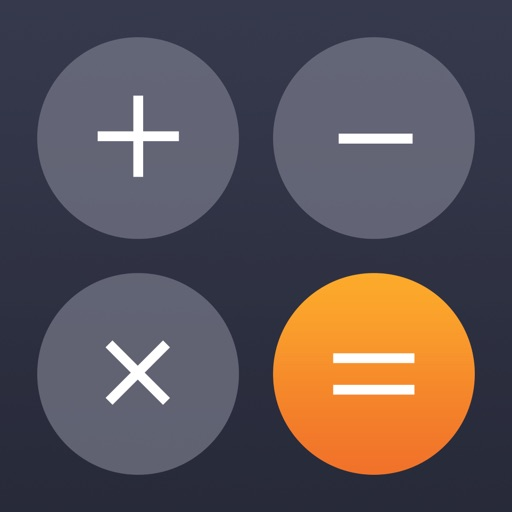## Calculator combines the Standard & Scientific calculator, with extensive & easy functionality options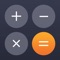# Calculator‘

by Impala Studios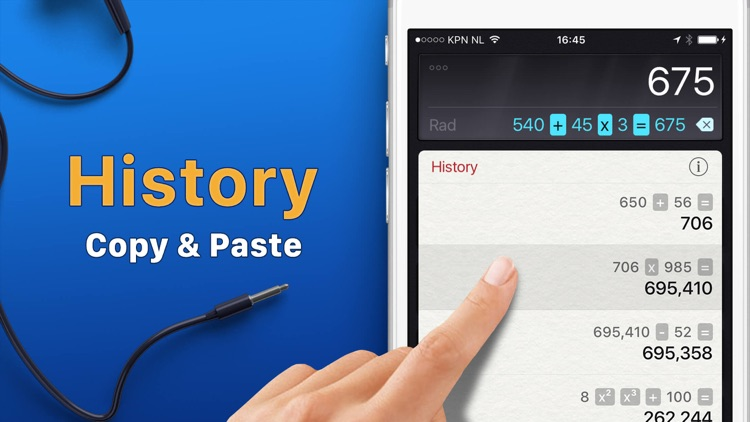Calculator combines the Standard & Scientific calculator, with extensive & easy functionality options. Use the new Retro Theme now and go back to vintage in time ; )### App Details

Version
3.4.11
Rating
(210999)
Size
92Mb
Genre
Utilities Education
Last updated
May 13, 2020
Release date
December 21, 2011

### App Screenshots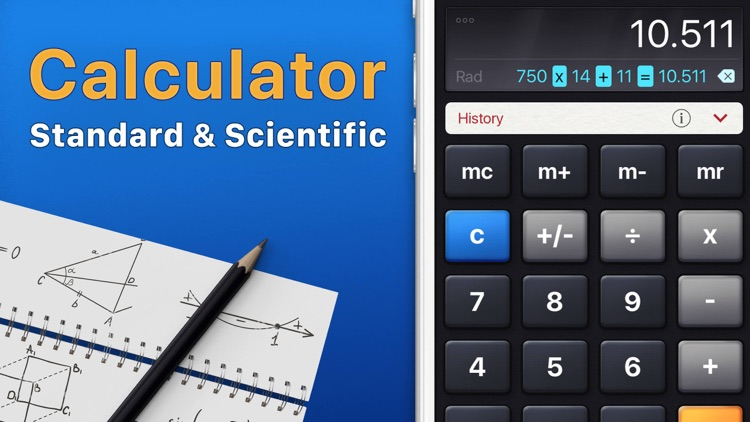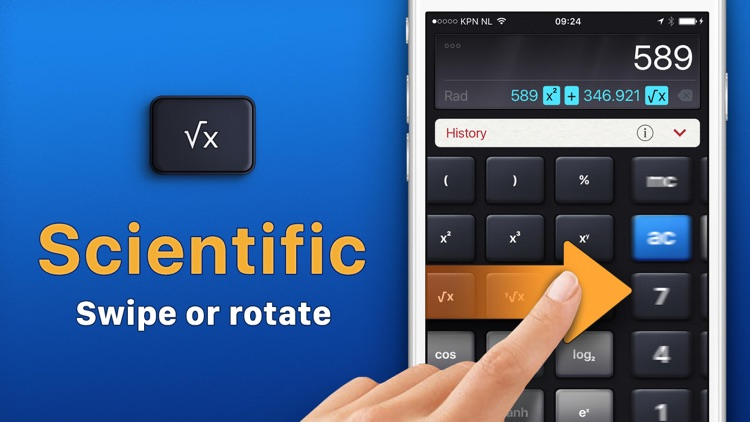### App Store Description

Calculator combines the Standard & Scientific calculator, with extensive & easy functionality options. Use the new Retro Theme now and go back to vintage in time ; )

Calculator is Fast, Accurate and Beautiful, it's everything you need right in the palm of your hand!

WHY YOU'LL LOVE CALCULATOR
• Basic & Scientific Calculations
• History Tape: Copy & Share
• Edit Equation
• Calculate with Degrees or Radians
• Memory
• Retro Theme
• Set the Number of Decimals
• Dark Mode
• Support for iPad Split View

EDIT EQUATION
You can edit both digits and operators of the current equation.

LIST OF MATHEMATICAL OPERATIONS
Square, Cubic Exponent, Nth Exponent
Square Root, Cubic Root, Nth Root
Engineering Exponent, Factorial
Power of Two, Power of Ten, Power of E
Natural Logarithm, Common Logarithm, Binary Logarithm
Sine, Cosine, Tangent
Arc Sine, Arc Cosine, Arc Tangent
Hyperbolic Sine, Hyperbolic Cosine, Hyperbolic Tangent
Hyperbolic Arc Sine, Hyperbolic Arc Cosine, Hyperbolic Arc Tangent
Multiplicative Inverse, Random Number Input